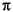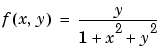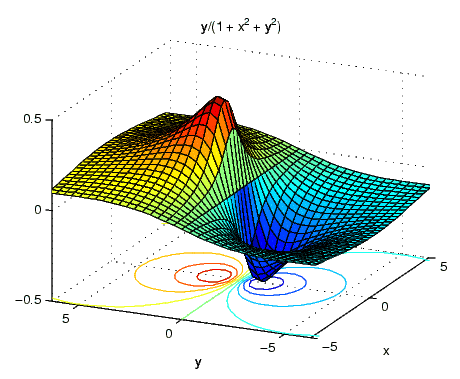MATLAB Function Referenceezsurfc

Easy to use combination surface/contour plotter

Syntax

• ```ezsurfc(fun)
ezsurfc(fun,domain)
ezsurfc(funx,funy,funz)
ezsurfc(funx,funy,funz,[smin,smax,tmin,tmax]) or
ezsurfc(funx,funy,funz,[min,max])
ezsurfc(...,n)
ezsurfc(...,'circ')
ezsurfc(axes_handle,...)
h = ezsurfc(...)
```

Description

`ezsurfc(fun)` creates a graph of `fun(x,y)` using the `surfc` function. The function `fun` is plotted over the default domain: -2< `x` < 2, -2< `y` < 2.

`fun` can be a function handle for an M-file function or an anonymous function (see Function Handles and Anonymous Functions) or a string (see the Remarks section).

```ezsurfc(fun,domain) ``` plots `fun` over the specified `domain`. `domain` can be either a 4-by-1 vector [`xmin`, `xmax`, `ymin`, `ymax`] or a 2-by-1 vector [`min`, `max`] (where `min` < `x` < `max`, `min` < `y` < `max`).

```ezsurfc(funx,funy,funz) ``` plots the parametric surface `funx(s,t)`, `funy(s,t)`, and f`unz(s,t)` over the square: -2< `s` < 2, -2< `t` < 2.

```ezsurfc(funx,funy,funz,[smin,smax,tmin,tmax]) or ezsurfc(funx,funy,funz,[min,max]) ``` plots the parametric surface using the specified domain.

```ezsurfc(...,n) ``` plots f over the default domain using an `n`-by-`n` grid. The default value for `n` is 60.

```ezsurfc(...,'circ') ``` plots f over a disk centered on the domain.

```ezsurfc(axes_handle,...) ``` plots into the axes with handle `axes_handle` instead of the current axes (`gca`).

```h = ezsurfc(...) ``` returns the handles to the graphics objects in `h`.

Remarks

Passing the Function as a String

Array multiplication, division, and exponentiation are always implied in the expression you pass to `ezsurfc`. For example, the MATLAB syntax for a surface/contour plot of the expression

• ```sqrt(x.^2 + y.^2);
```

is written as

• ```ezsurfc('sqrt(x^2 + y^2)')
```

That is, `x^2` is interpreted as `x.^2` in the string you pass to `ezsurfc`.

If the function to be plotted is a function of the variables u and v (rather than x and y), then the domain endpoints `umin`, `umax`, `vmin`, and `vmax` are sorted alphabetically. Thus, `ezsurfc('u^2 - v^3',[0,1],[3,6])` plots u2 - v3 over 0 < u < 1, 3 < v < 6.

Passing a Function Handle

Function handle arguments must point to functions that use MATLAB syntax. For example, the following statements define an anonymous function and pass the function handle `fh` to `ezsurfc`.

• ```fh = @(x,y) sqrt(x.^2 + y.^2);
ezsurf(fh)
```

Note that when using function handles, you must use the array power, array multiplication, and array division operators (`.^, .*, ./`) since `ezsurfc` does not alter the syntax, as in the case with string inputs.

Passing Additional Arguments

If your function has additional parameters, for example `k` in `myfun`:

• ```function z = myfun(x,y,k1,k2,k3)
z = x.*(y.^k1)./(x.^k2 + y.^k3);
```

then you can use an anonymous function to specify that parameter:

• ```ezsurfc(@(x,y)myfun(x,y,2,2,4))
```

Examples

Create a surface/contour plot of the expression

•over the domain -5 < x < 5, -2*pi < y < 2*pi, with a computational grid of size 35-by-35:

• ```ezsurfc('y/(1 + x^2 + y^2)',[-5,5,-2*pi,2*pi],35)
```

Use the mouse to rotate the axes to better observe the contour lines (this picture uses a view of azimuth = -65.5 and elevation = 26).See Also

`ezmesh`, `ezmeshc`, `ezsurf`, `function_handle`, `surfc`

Function Plots for related functions

© 1994-2005 The MathWorks, Inc.